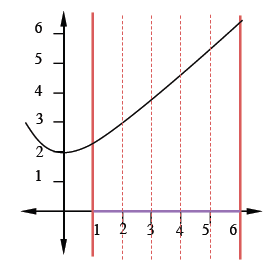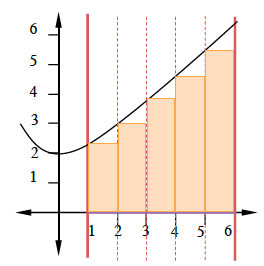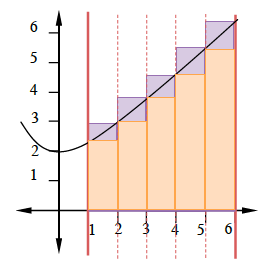### Home > PC > Chapter 2 > Lesson 2.3.4 > Problem2-112

2-112.
1. Let f(x) =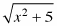. Homework Help ✎

1. Graph the function for 1 ≤ x ≤ 6.

2. Find upper and lower approximations for the area by using five right-endpoint and five left-endpoint rectangles.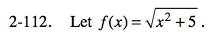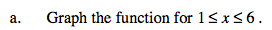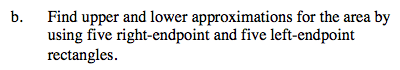L = 1(f(1)+…+ f(5))

R = 1(f(2)+…+ f(6) )